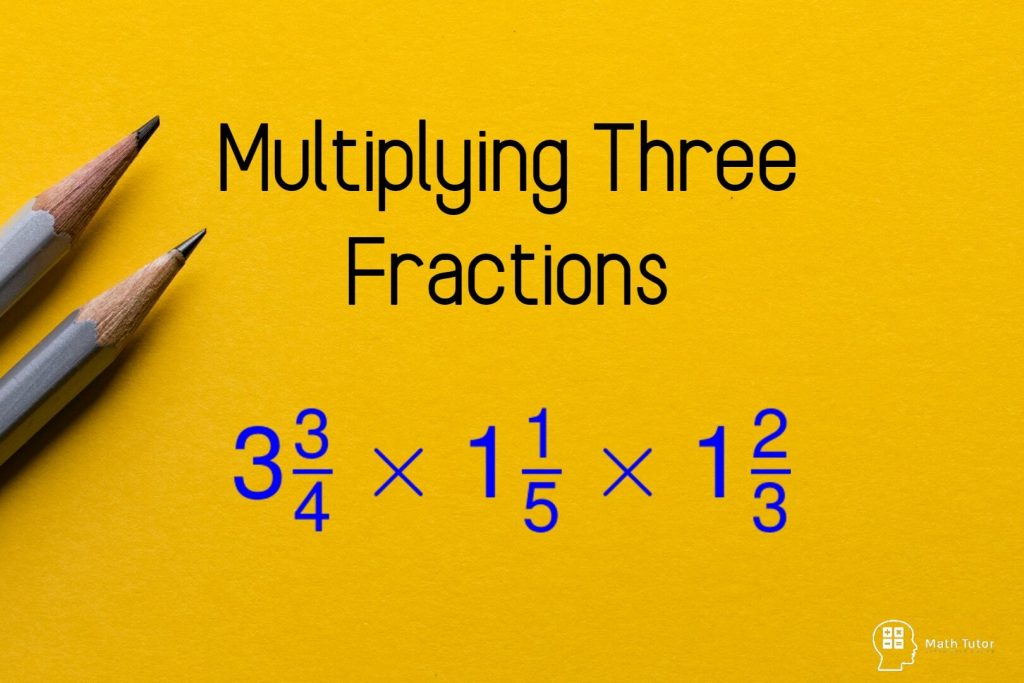# Numbers

Numbers## Multiplying Three Fractions

In this lesson, we are going to learn how to multiplying three fractions.We have discussed how to multiply fractions from basics in another lesson. You can visit the following link to view the previous lesson.How to Multiply Fractions Multiplying Three Fractions Multiplying three fractions seems a little more complicated than multiplying two fractions. But the …## Trigonometric Identities

A Trigonometric identity or trig identity is an identity that contains the trigonometric functions sine(sin), cosine(cos), tangent(tan), cotangent(cot), secant(sec), or cosecant(csc). Trigonometric identities can use to: Simplify trigonometric expressions. Solve trigonometric equations. Prove that one trigonometric expression is equivalent to another so that we can replace the first expression by the second expression. The following …The addition of fractions can discuss in a few categories Unit fraction addition Improper fraction addition Mixed number addition There is only one golden rule that we have to follow when the adding  fractions, There should be a common denominator between the fractions Unit Fraction Addition Example 01: Like Denominators Yellow color area : Green …## Mixed Numbers to Improper Fractions Conversion

In this article, we are going to discuss how to convert Mixed Numbers to Improper Fractions. Before moving into that part, let’s recall what mixed numbers and improper fractions are. A whole pizza and exactly half of an identical pizza shown in the figure. Therefore the total amount of pizza in the picture is 1 …## How to Multiply Fractions in 3 Steps

How to Multiply Fractions Multiply fractions can discuss under three main categories. Multiplication of proper fractions  Multiplication of improper fractions Multiplication of a mixed numbers Multiplication of Proper Fractions A proper fraction is a fraction that is less than one, with the numerator less than the denominator. Example : To multiply proper fractions you have …